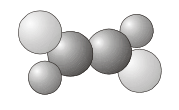# 2: Symmetry Operations and Symmetry Elements

•• Contributed by Claire Vallance
• Professor of Physical Chemistry (Department of Chemistry) at University of Oxford

A symmetry operation is an action that leaves an object looking the same after it has been carried out. For example, if we take a molecule of water and rotate it by 180° about an axis passing through the central O atom (between the two H atoms) it will look the same as before. It will also look the same if we reflect it through either of two mirror planes, as shown in the figure below.Each symmetry operation has a corresponding symmetry element, which is the axis, plane, line or point with respect to which the symmetry operation is carried out. The symmetry element consists of all the points that stay in the same place when the symmetry operation is performed. In a rotation, the line of points that stay in the same place constitute a symmetry axis; in a reflection the points that remain unchanged make up a plane of symmetry.

The symmetry elements that a molecule may possess are:

1. $$E$$ - the identity. The identity operation consists of doing nothing, and the corresponding symmetry element is the entire molecule. Every molecule has at least this element.
2. $$C_n$$ - an $$n$$-fold axis of rotation. Rotation by $$360°/n$$ leaves the molecule unchanged. The $$H_2O$$ molecule above has a $$C_2$$ axis. Some molecules have more than one $$C_n$$ axis, in which case the one with the highest value of $$n$$ is called the principal axis. Note that by convention rotations are counterclockwise about the axis.
3. $$\sigma$$ - a plane of symmetry. Reflection in the plane leaves the molecule looking the same. In a molecule that also has an axis of symmetry, a mirror plane that includes the axis is called a vertical mirror plane and is labeled $$\sigma_v$$, while one perpendicular to the axis is called a horizontal mirror plane and is labeled $$\sigma_h$$. A vertical mirror plane that bisects the angle between two $$C_2$$ axes is called a dihedral mirror plane, $$\sigma_d$$.
4. $$i$$ - a center of symmetry. Inversion through the center of symmetry leaves the molecule unchanged. Inversion consists of passing each point through the center of inversion and out to the same distance on the other side of the molecule. An example of a molecule with a center of inversion is shown below.1. $$S_n$$ - an n-fold improper rotation axis (also called a rotary-reflection axis). The rotary reflection operation consists of rotating through an angle $$360°/n$$ about the axis, followed by reflecting in a plane perpendicular to the axis. Note that $$S_1$$ is the same as reflection and $$S_2$$ is the same as inversion. The molecule shown above has two $$S_2$$ axes.

The identity $$E$$ and rotations $$C_n$$ are symmetry operations that could actually be carried out on a molecule. For this reason they are called proper symmetry operations. Reflections, inversions and improper rotations can only be imagined (it is not actually possible to turn a molecule into its mirror image or to invert it without some fairly drastic rearrangement of chemical bonds) and as such, are termed improper symmetry operations.

Axis Definitions

Conventionally, when imposing a set of Cartesian axes on a molecule (as we will need to do later on in the course), the $$z$$ axis lies along the principal axis of the molecule, the $$x$$ axis lies in the plane of the molecule (or in a plane containing the largest number of atoms if the molecule is non-planar), and the $$y$$ axis makes up a right handed axis system.